Next: Exercises Up: Axisymmetric Incompressible Inviscid Flow Previous: Flow Around a Submerged

# Flow Around a Submerged Prolate Spheroid

Consider the conformal map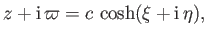(7.142)

where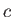is real and positive. It follows that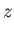(7.143)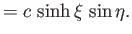(7.144)

Let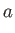(7.145)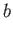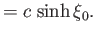(7.146)

Thus, in the meridian plane, the curve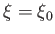corresponds to the ellipse(7.147)

We conclude that the surfaceis an prolate spheroid (i.e., the three-dimensional surface obtained by rotating an ellipse about a major axis) of major radius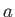and minor radius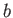. The constraints (7.87) and (7.90) yield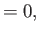(7.148)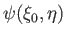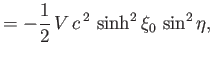(7.149)

respectively. Setting, and substituting into the governing equation, (7.86), we obtain(7.150)

The solution that satisfied the constraint (7.148) is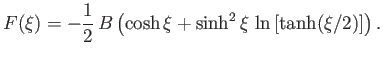(7.151)

Letbe the eccentricity of the spheroid. Thus,,,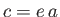, and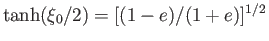. The constraint (7.149) yields(7.152)

Hence,(7.153)

Finally, from Equation (7.84),(7.154)

which can be integrated to give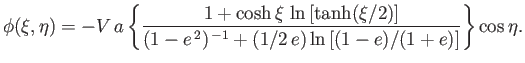(7.155)

It is easily demonstrated that(7.156)

and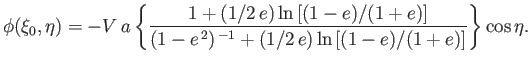(7.157)

Thus,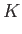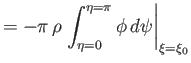(7.158)

It follows that the added mass of the spheroid is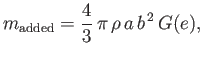(7.159)

where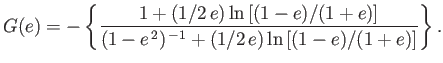(7.160)

is a monotonic function that takes the valuewhen, and asymptotes to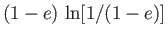as.Next: Exercises Up: Axisymmetric Incompressible Inviscid Flow Previous: Flow Around a Submerged
Richard Fitzpatrick 2016-03-31